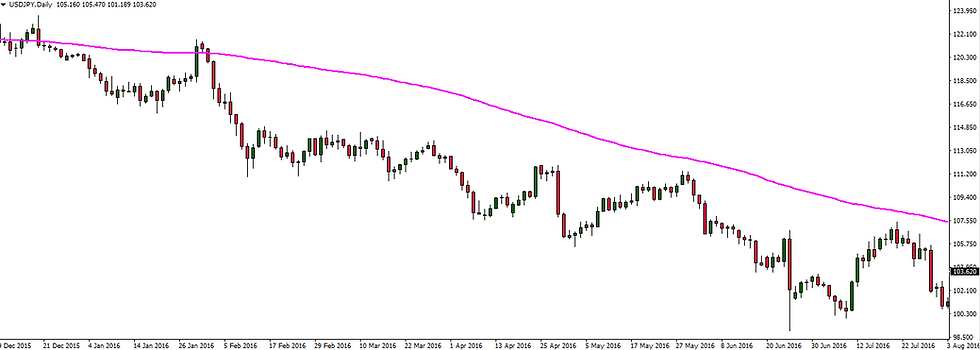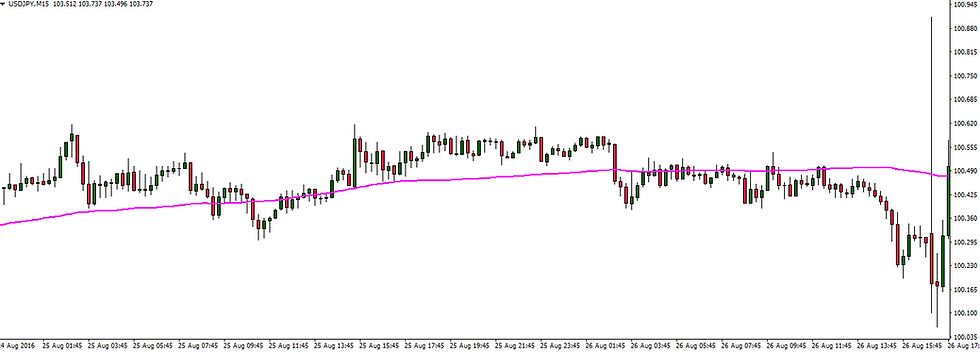top of page

# Forex 101 - Moving Averages

Moving averages are the most commonly used technical indicator in price chart analysis. They are used by a vast amount of Forex retail traders and professional institutions and are commonly respected amongst top analysts and financial market media broadcasters, such as Bloomberg and Thomson Reuters. This post will give you a simple but detailed explanation about moving averages and how you can use them profitably in your Forex trading.

What are Moving Averages?

Just like all technical indicators, moving averages are used to help predict future price movement (more on that later). Moving averages use the closing prices of a particular time-frame and plot the average closing price on the chart. For example, if I was using the 1 hour time-frame and had a moving average set at the period of 15, the indicator formula would take the last 15 hours of closing price data and mark the average closing price on the chart. This is done with every set of 15 hours and all the averages closing prices are joined by a line, creating a sort of line graph on the price chart. Perhaps a better way to explain this is to show you a price chart with moving averages set at a period of 15, 50 and 75...The image may seem a bit daunting but by the end of this post, you should have a much better understanding of moving averages and know how to use them in your Forex trading.

Types of Moving Averages?

In technical analysis, there are many types of moving averages but the 2 most common types used by traders and investors are Simple Moving Averages (SMA's) and Exponential Moving Averages (EMA's).

Simple Moving Averages

SMA's are calculated as suggested in the section above; the formula takes a block of closing prices (the amount being determined by the period of the moving average), the average closing price is calculated, the average price is then marked on the price chart.

Exponential Moving Averages

EMA's are calculated in a similar fashion to SMA's but they put more weight/emphasis on more recent closing price data.

The chart below shows the difference between a 60 period SMA and a 60 period EMA. Do you notice how the EMA is slightly more aggressive, even though the period setting is the same? This is because of the added weight in the EMA indicator formula.How to use Moving Averages

Moving averages can be used in a variety of ways. Below are some of the most popular ways to use moving averages in technical analysis.

Trend Direction

Moving averages can be used to determine trend/price direction. This is generally done in 2 ways. The 1st being that the direction of the moving average indicates price direction. Look at the price chart below. It is a daily chart of the USDJPY. Do you notice that the moving average is bearish(moving down) and so is price?In the next Forex chart, the moving average is moving sideways and so is price (USDJPY 15 Minute Chart).Another way to determine price direction when using moving averages is to analyse where price is compared to the moving average. Going back to the USDJPY daily chart, you should notice that the Japanese Candlesticks are clearly opening and closing below the moving average. This suggests that price could continue to downtrend. If the candles were clearly opening and closing above the moving average, this could suggest that price may uptrend. When price frequently changes from being below the moving average and being above the moving average, this suggests that price is indecisive and could be ranging. You should be able to notice this on the USDJPY 15 minute chart above.

Price Reversal

Having a faster (lower period) moving average and a slower (higher period) moving average on a Forex price chart can help to signal price reversal. When one moving average crosses the other moving average, this can suggest that price is about to reverse. These crosses are often referred to as Moving Average Crosses. Look at the Forex price chart below. We have highlighted each time the moving averages cross. Do you notice that the moving average crosses act as a signal for a change in price direction? The moving average periods are 15 and 25.Dynamic Support and Resistance

Moving averages can also provide areas of market support and resistance (if you need a refresher on support and resistance, please check out our Forex Support and Resistance page). As price reaches commonly used period moving averages, price can reverse i.e find support or resistance. Below is a chart of the GBPUSD. Each time price reaches the moving average and finds either support or resistance, this has been highlighted.What Moving Averages should you use?

One of the many things I love about Forex trading is that you can be as creative as you want to be. There are no boundaries on what period your moving averages should be, what indicators to use (if any), what time-frame to trade, etc. The most popular moving average settings though, are the 7, 21, 50, 100 and 200 periods.

Was this post beneficial? Check out our other Forex 101 Indicator posts.

Tags:

bottom of page